Biogeosciences, 19, 3575–3593, 2022
https://doi.org/10.5194/bg-19-3575-2022
Biogeosciences, 19, 3575–3593, 2022
https://doi.org/10.5194/bg-19-3575-2022
Research article | 03 Aug 2022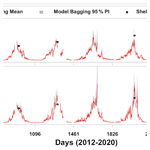# Hydrodynamic and biochemical impacts on the development of hypoxia in the Louisiana–Texas shelf – Part 2: statistical modeling and hypoxia prediction

Hydrodynamic and biochemical impacts on the development of hypoxia in the Louisiana–Texas shelf – Part 2: statistical modeling and hypoxia prediction
Yanda Ou1, Bin Li2, and Z. George Xue1,3,4 Yanda Ou et al.
• 1Department of Oceanography & Coastal Sciences, Louisiana State University, Baton Rouge, LA 70803, USA
• 2Department of Experimental Statistics, Louisiana State University, Baton Rouge, LA 70803, USA
• 3Center for Computation & Technology, Louisiana State University, Baton Rouge, LA 70803, USA
• 4Coastal Studies Institute, Louisiana State University, Baton Rouge, LA 70803, USA

Correspondence: Z. George Xue (zxue@lsu.edu)

Abstract

This study presents a novel ensemble regression model for forecasts of the hypoxic area (HA) in the Louisiana–Texas (LaTex) shelf. The ensemble model combines a zero-inflated Poisson generalized linear model (GLM) and a quasi-Poisson generalized additive model (GAM) and considers predictors with hydrodynamic and biochemical features. Both models were trained and calibrated using the daily hindcast (2007–2020) by a three-dimensional coupled hydrodynamic–biogeochemical model embedded in the Regional Ocean Modeling System (ROMS). Compared to the ROMS hindcasts, the ensemble model yields a low root-mean-square error (RMSE) (3256 km2), a high R2 (0.7721), and low mean absolute percentage biases for overall (29 %) and peak HA prediction (25 %). When compared to the shelf-wide cruise observations from 2012 to 2020, our ensemble model provides a more accurate summer HA forecast than any existing forecast models with a high R2 (0.9200); a low RMSE (2005 km2); a low scatter index (15 %); and low mean absolute percentage biases for overall (18 %), fair-weather summer (15 %), and windy-summer (18 %) predictions. To test its robustness, the model is further applied to a global forecast model and produces HA prediction from 2012–2020 with the adjusted predictors from the HYbrid Coordinate Ocean Model (HYCOM). In addition, model sensitivity tests suggest an aggressive riverine nutrient reduction strategy (92 %) is needed to achieve the HA reduction goal of 5000 km2.

Share
1 Introduction

The Louisiana–Texas (LaTex) shelf has become a center of hypoxia (bottom dissolved oxygen, DO < 2 mg L−1) study since the 1980s (e.g., Rabalais et al., 2002, 2007a; Justić and Wang, 2014). Regular mid-summer shelf-wide cruises documented that the area and volume of hypoxic bottom water could reach up to 23 000 km2 and 140 km3, respectively (Rabalais and Turner, 2019; Rabalais and Baustian, 2020). The aquatic environments, fisheries, and coastal economies are under threat of recurring hypoxia in summer (Chesney and Baltz, 2001; Craig and Bosman, 2013; de Mutsert et al., 2016; LaBone et al., 2021; Rabalais and Turner, 2019; Rabotyagov et al., 2014; Smith et al., 2014). For example, habitats of some fish species (e.g., croaker and brown shrimp) shift to offshore hypoxic edges (Craig and Crowder, 2005; Craig, 2012) during summer hypoxia events, which may impact organism energy budgets and trophic interactions (Craig and Crowder, 2005; Hazen et al., 2009). The horizontal displacement of habitats of brown shrimp in summer can also lead to changes in the distribution of Gulf of Mexico shrimp fleets (Purcell et al., 2017). Although an action plan has been launched by the Mississippi River/Gulf of Mexico Hypoxia Task Force to control the size of the mid-summer hypoxic zone below 5000 km2 in a 5-year running average since 2001 (Mississippi River/Gulf of Mexico Watershed Nutrient Task Force, 2001, 2008), extents of the hypoxic area have experienced no significant decreases in recent decades (Del Giudice et al., 2020). An accurate prediction of the hypoxic area is urgently needed for coastal managers and the fishery industry.

These above-mentioned models share some similar drawbacks. (1) The effects of water column stratification are considered only implicitly by the associated wind speeds, water transport, and riverine nutrient loads (usually correlated to river discharges), although stratification is documented as a crucial factor in regulating HA variability. (2) Forecasts of the predictors are usually limited, which restricts some of these seasonal models to pseudo ones. (3) Most models are only capable of capturing interannual HA variability and are not reliable in summers when winds are strong. According to the hindcast results by our three-dimensional coupled hydrodynamic–biogeochemical model described in the accompanying paper (Part 1), strong wind events bring considerable uncertainties to monthly and daily variabilities in HA. In this study we aim to provide a novel HA prediction method that considers both stratification and biochemical effects. Our new model aims to produce daily HA forecasts based on selected predictors' forecasts with a minimum computational cost. The rest of the paper is organized as follows. Detailed descriptions of methods and data are given in Sect. 2. The implementations of generalized linear models (GLMs), generalized additive models (GAMs), and an independent model application using a global forecast product (HYbrid Coordinate Ocean Model, HYCOM; Bleck and Boudra, 1981; Bleck, 2002) are given in Sect. 3. Comparisons against existing forecast models and recommendations on nutrient reduction strategy are given in Sect. 4.

2 Methods

## 2.1 Data preparation

We adapted a three-dimensional coupled hydrodynamic–biogeochemical model embedded in the framework of the Regional Ocean Modeling System (ROMS) on the platform of Coupled Ocean–Atmosphere–Wave–Sediment Transport (COAWST) modeling system (Warner et al., 2010) to the Gulf of Mexico (GoM; Gulf–COAWST, for detailed descriptions, validations, and results of the numerical model, see Part 1). Numerical hindcasts (hereafter denoted as ROMS hindcasts or ROMS simulations) are output daily from 1 January 2007 to 26 August 2020 and spatially averaged over the LaTex shelf extending from the west of Mississippi River mouth to 95 W with water depths ranging from 6 to 50 m (color shaded region in Fig. A1b).

### 2.1.1 Hydrodynamic-related predictors

Both water stratification and bottom biochemical processes modulate the variability in bottom DO concentration in the LaTex shelf. Potential energy anomaly (PEA, in J m−3) is introduced as an estimate of water column stratification according to

$\begin{array}{}\text{(1)}& \mathrm{PEA}=\frac{\mathrm{1}}{H}{\int }_{-h}^{\mathit{\eta }}\left(\stackrel{\mathrm{‾}}{\mathit{\rho }}-\mathit{\rho }\right)gz\mathrm{d}z,\end{array}$

where ρ is the water density profile (estimated by water temperature and salinity profiles) over the water column of depth $H=h+\mathit{\eta }$, h is the location of the bed, η is water surface elevation, g is the gravitational acceleration (9.8 m s−2), z is the vertical axis, and $\stackrel{\mathrm{‾}}{\mathit{\rho }}$ is the depth-integrated water density given by $\stackrel{\mathrm{‾}}{\mathit{\rho }}=\frac{\mathrm{1}}{H}{\int }_{-h}^{\mathit{\eta }}\mathit{\rho }\mathrm{d}z$ (Simpson and Hunter, 1974, 1978; Simpson, 1981; Simpson and Bowers, 1981). The PEA represents the amount of energy per volume required to homogenize the entire water column (Simpson and Hunter, 1974). Thus, a greater PEA value represents a more stratified water column. As a river-dominated area, water stratification in the LaTex shelf is highly affected by freshwater-induced buoyancy from the Mississippi and Atchafalaya rivers. Sea surface salinity (SSS) is a good proxy for representing the distribution and variability in river freshwater across the shelf. Indeed, the correlation of regionally averaged PEA and SSS is significantly high as 0.87 (p<0.001; Fig. 1a), which emphasizes the importance of freshwater-induced stratification. Therefore, we considered SSS as another candidate predictor besides PEA.

Surface heating and wind mixing are two other factors that influence water stratification (Simpson, 1981). The tidal effects considered in Simpson (1981) are neglected here due to the relatively weaker contribution in stratification in the shelf when compared to the effects of rivers and winds. The two mixing terms are quantified as follows:

$\begin{array}{}\text{(2)}& \frac{\mathrm{d}\left(\mathrm{PEA}\right)}{\mathrm{d}t}=\frac{\mathit{\alpha }g}{\mathrm{2}c}Q-\mathit{\delta }{k}_{\mathrm{a}}{\mathit{\rho }}_{\mathrm{a}}{W}^{\mathrm{3}},\end{array}$

where Q is the rate of surface heat input, α is the volume expansion coefficient, c is water specific heat capacity, δ is a coefficient of wind mixing, ka is the drag coefficient, ρa is the density of humid air near the sea surface, and W is the wind speed near the sea surface. The first term on the right-hand side of Eq. (2) represents the rate of change in water stratification due to surface heating, while the second term is the rate of working by wind stress contributing negatively to water stratification. Therefore, the heat-induced change in PEA is proportional to surface heat input, which is

$\begin{array}{}\text{(3)}& \mathrm{d}\left(\mathrm{PEA}{\right)}_{\mathrm{heat}}\propto Q.\end{array}$

The total net heat flux, a sum of net shortwave and net longwave radiation flux, is derived from the National Centers for Environmental Prediction (NCEP) Climate Forecast System Reanalysis (CFSR) 6-hourly products (Saha et al., 2010, 2011) in this study. The term Q is added to the candidate list of predictors and is denoted as PEAheat (heat-induced PEA changes) for simplification (Fig. 1a).

Daily variability in the term (δkaρaW3) is dominated by that of W3, since the ρa fluctuates much less than the W3 on a daily scale (Fig. A2). We obtained ρa according to (Picard et al., 2008):

$\begin{array}{}\text{(4)}& {\mathit{\rho }}_{\mathrm{a}}=\frac{p{M}_{\mathrm{d}}}{ZRT}\left[\mathrm{1}-{x}_{\mathrm{v}}\left(\mathrm{1}-\frac{{M}_{\mathrm{v}}}{{M}_{\mathrm{d}}}\right)\right],\end{array}$

where p represents the absolute air pressure, Md (28.96546 g mol−1) is the molar mass of dry air, Mv (18.01528 g mol−1) is the molar mass of water vapor, Z indicates compressibility, R (8.314472 J mol−1 K−1) is the molar gas constant, T is thermodynamic temperature, and xv is the mole fraction of water vapor. We assumed that air parcels at the sea surface are ideal gases (Z=1) and are always saturated with water vapor. Thus, xv is a function of the absolute air pressure (p) and saturation vapor pressure of water (psat) and can be calculated as follows:

$\begin{array}{}\text{(5)}& {x}_{\mathrm{v}}=\frac{{p}_{\mathrm{sat}}}{p}.\end{array}$

According to the adjusted Tetens equation (Murray, 1967; Monteith and Unsworth, 2014), psat (in Pa) can be estimated by

$\begin{array}{}\text{(6)}& {p}_{\mathrm{sat}}=\mathrm{611}{e}^{\frac{\mathrm{17.27}\left(T-\mathrm{237.3}\right)}{T-{T}^{\prime }}},\end{array}$

where ${T}^{\prime }=\mathrm{36}$ K. Substituting Eqs. (5)–(6) for Eq. (4) with the assumption of Z=1, we obtained air density as a function of both air pressure and air temperature in the following:

$\begin{array}{}\text{(7)}& {\mathit{\rho }}_{\mathrm{a}}={\mathit{\rho }}_{\mathrm{a}}\left(T,p\right)=\frac{p{M}_{\mathrm{d}}}{RT}\left[\mathrm{1}-\frac{\mathrm{611}}{p}\left(\mathrm{1}-\frac{{M}_{\mathrm{v}}}{{M}_{\mathrm{d}}}\right){e}^{\frac{\mathrm{17.27}\left(T-\mathrm{237.3}\right)}{T-{T}^{\prime }}}\right].\end{array}$

ρa is then estimated using sea surface air pressure and air temperature 2 m above the sea surface provided by NCEP CFSR 6-hourly products. The correlation of daily ρaW3 and W3 (provided by NCEP CFSR 6-hourly products) is significantly high as 0.9988 (p<0.001, Fig. A2), emphasizing the importance of term W3 in controlling the daily variability in wind-induced PEA changes over the shelf. We, thus, approximated the relationship as

$\begin{array}{}\text{(8)}& \mathrm{d}\left(\mathrm{PEA}{\right)}_{\mathrm{wind}}\propto {W}^{\mathrm{3}}.\end{array}$

The term W3 is introduced as another candidate predictor and is denoted as PEAwind (wind-induced PEA changes) for simplification (Fig. 1a).

### 2.1.2 Biochemical-related predictors

$\begin{array}{}\text{(9)}& \mathrm{SOC}={\mathrm{PON}}_{\mathrm{sed}}\cdot \mathrm{VP}\mathrm{2}{\mathrm{N}}_{\mathrm{0}}\cdot {e}^{{K}_{\mathrm{P}\mathrm{2}\mathrm{N}}\cdot {T}_{\mathrm{b}}},\text{(10)}& \begin{array}{rl}\mathrm{SOCalt}=& \text{Mississippi River inorganic}\\ & \text{nitrogen loads}\phantom{\rule{0.125em}{0ex}}\left(\text{led\hspace{0.17em} by\hspace{0.17em} 44\hspace{0.17em}d}\right)\phantom{\rule{0.125em}{0ex}}\cdot {e}^{\mathrm{0.0693}{T}_{\mathrm{b}}},\end{array}\end{array}$

where VP2N0 is a constant representing the decomposition rates of sedimentary particulate organic nitrogen PONsed at 0 C, KP2N is a constant (0.0693 C−1) indicating temperature coefficients for decomposition of PONsed, and Tb is bottom water temperature (in C). The Q10 (2 given the above chosen coefficients; van 't Hoff and Lehfeldt, 1899; Reyes et al., 2008) assumption is applied to mimic the aerobic decomposition rate of PONsed. Along with SOCalt, the temperature-dependent decomposition rate ${e}^{\mathrm{0.0693}\cdot {T}_{\mathrm{b}}}$ is also considered a candidate predictor in statistical models and is denoted as DCPTemp for simplification.

### 2.1.3 HA estimation

As listed in Table 1, six candidate predictors are considered in the statistical models including four stratification-related variables (PEA, SSS, PEAheat, and PEAwind) and two bottom biochemical variables (SOCalt and DCPTemp). The correlation matrix (Fig. 1a) indicates that multicollinearity may become a problem in regression models, since linear correlations among some predictors are significantly high, e.g., 0.74 (p<0.001) between PEA and SOCalt and 0.87 (p<0.001) between PEA and SSS. The multicollinearity can violate the assumption that predictors are independent. It can lead to difficulties in individual coefficient tests and numerical instability (Siegel and Wagner, 2022). The frequency distribution of HA (Fig. 1b) illustrates that the response variable is highly skewed to the right with  42 % of samples (2081 out of 4943) being exactly zero. HA is estimated by the number of hypoxia cells (ROMS computational cells reaching hypoxic conditions) times a nearly constant value (area of the computational cell), which is 25.56 ± 0.17 km2 (mean ± 1 SD). Thus, HA can be estimated by the number of grid cells when the Poisson and negative binomial regression models are applied. However, the great portion of zero samples leads to overdispersion (magnitude of variance magnitude of mean, i.e., 45 730 441  4507) and zero-inflated problems (Lambert, 1992). The overdispersion issue violates the mean-variance equality assumption employed in regular Poisson regression models, while zero-inflated problems can weaken the model performances.

Table 1Description of daily response variable and candidate predictors. The data cover a time range from 1 January 2007 to 26 August 2020. Prescribed min and max values are used for min–max normalization.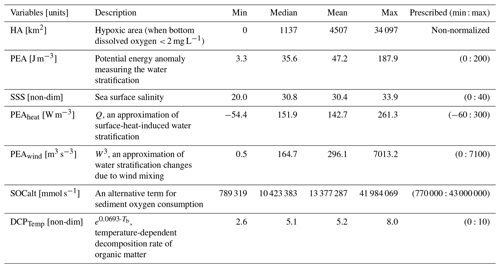## 2.2 Data pre-processes

We first spatially averaged ROMS-derived predictors (daily) over the LaTex shelf (color-shaded area in Fig. A1b) and then applied the min–max normalization (Eq. 11) to the one-dimensional time series. Predictive models can be beneficial from the min–max normalization when applying to a new dataset, since the method guarantees that the normalized predictors from different datasets range from 0 to 1 as the minimum and maximum values are prescribed. Note that the response is not normalized.

$\begin{array}{}\text{(11)}& {X}_{\mathrm{nor}}=\frac{{X}_{\mathrm{org}}-{\mathrm{min}}_{\mathrm{prescribed}}}{\left({\mathrm{max}}_{\mathrm{prescribed}}-{\mathrm{min}}_{\mathrm{prescribed}}\right)},\end{array}$

where Xnor, Xorg, minprescribed, and maxprescribed represent the normalized value, original value, prescribed minimum, and prescribed maximum, respectively. The daily samples are then split into a training set (for model construction) accounting for 80 % of the total samples and a test set (for assessment of model performances) accounting for the remaining 20 %. To maintain the HA distribution in both sets, a stratified random resampling method is applied in different HA intervals individually. For example, 80 % of samples with HA = 0 are chosen randomly for the training set out of all daily samples with HA = 0, while the rest of the samples with HA = 0 are grouped into the test set. HA = 0 is the first interval to which the resampling process is applied, while the remaining samples are split at intervals of 5000 km2. However, the distribution of HA from each year is similar with a right-skewed structure and numerous zero values. Thus, even through random processes, both the training and test sets contain samples from each year including samples with non-peak and peak HA. This splitting method increases the model applicability and provides a comprehensive assessment of prediction performances on both non-peak and peak HA.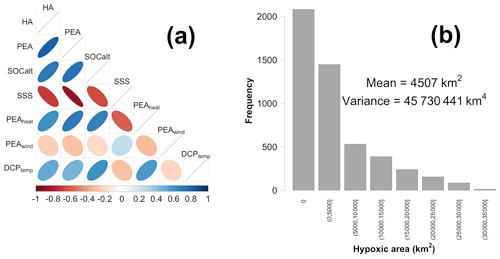Figure 1(a) A correlation matrix of the response variable and candidate predictors and (b) the frequency distribution of HA. Data are provided daily from 1 January 2007 to 26 August 2020.

## 2.3 Model skill assessment

The R2, root-mean-square error (RMSE), mean absolute percentage bias (MAPB), and scatter index (SI; Zambresky, 1989) are used to assess the model performances in HA predictions. The SI is a normalized measure of error or a relative percentage of expected error with respect to the mean observation. The calculations of the statistics are given in Eqs. (12)–(15):

$\begin{array}{}\text{(12)}& {R}^{\mathrm{2}}=\mathrm{1}-\frac{{\sum }_{i=\mathrm{1}}^{N}{\left({P}_{i}-{O}_{i}\right)}^{\mathrm{2}}}{{\sum }_{i=\mathrm{1}}^{N}\left({P}_{i}-\stackrel{\mathrm{‾}}{O}{\right)}^{\mathrm{2}}},\text{(13)}& \mathrm{RMSE}=\sqrt{\frac{{\sum }_{i=\mathrm{1}}^{N}{\left({P}_{i}-{O}_{i}\right)}^{\mathrm{2}}}{N}},\text{(14)}& \mathrm{MAPB}=\frac{\mathrm{1}}{N}{\sum }_{i=\mathrm{1}}^{N}\left|\frac{{P}_{i-}{O}_{i}}{{O}_{i}}\right|×\mathrm{100}\phantom{\rule{0.125em}{0ex}}\mathit{%},\text{(15)}& \mathrm{SI}=\frac{\mathrm{RMSE}}{\stackrel{\mathrm{‾}}{O}}×\mathrm{100}\phantom{\rule{0.125em}{0ex}}\mathit{%},\end{array}$

where Pi and Oi represent the ith record of prediction and observation (or hindcast), while $\stackrel{\mathrm{‾}}{O}$ represents the average of all observed (or hindcast) records.

3 Model construction and results

## 3.1 Model built-up process

Several regression models are explored using the statistical programming language R. To find the “best” model balancing both model interpretability and prediction performance, a procedure is conducted for model selection (Fig. 2) and is summarized below. (1) Choose a regression model. (2) Apply an exhaustive best-subset searching approach to the chosen model. Models with possible combinations of candidate predictors from the ROMS training set are built. A 10-fold cross-validation (CV) method is applied to each model, yielding 10 RMSEs and 1 corresponding mean. The candidate predictors of PEA and SOCalt are forced into each subset. Thus, the number of fitted models with a subset size of k is $C\left(\mathrm{6}-\mathrm{2},k-\mathrm{2}\right)=\frac{\mathrm{4}\mathrm{!}}{\left(\mathrm{6}-k\right)\mathrm{!}\left(k-\mathrm{2}\right)\mathrm{!}}\phantom{\rule{0.125em}{0ex}},\phantom{\rule{0.125em}{0ex}}\mathrm{2}\le k\le \mathrm{6}$ (the total number of candidate predictors is 6). The optimal subset of this size is found as the one with the lowest mean CV RMSE among these models. The best subset is then obtained by comparing mean CV RMSEs of the optimal subsets of different sizes. (3) Steps (1)–(2) are repeated for the selected M candidate regression models. (4) Prediction performances of different models with the corresponding best subsets are assessed by the 10-fold CV RMSEs and bootstrap (1000 iterations) aggregating (i.e., bagging) ensemble algorithms. The bagging method builds the given model N (1000) times during each of which the given model is trained using different samples chosen randomly and repeatedly from the ROMS training set and is executed for HA prediction using samples in the ROMS test set. The ensemble means and ensemble 95 % prediction intervals (PIs) of forecast HA are given according to the prediction results in the 1000 iterations. The best model (model X in Fig. 2) is chosen according to the comparisons of the 10-fold CV RMSEs and the bagging results.

## 3.2 Generalized linear models (GLMs)

### 3.2.1 Regular GLMs and zero-inflated GLMs

The response variable can be treated as count data. Regular Poisson (function glm in R package stats version 3.6.2), quasi-Poisson (function glm in R package stats version 3.6.2), and negative binomial (function glm.nb in R package MASS version 7.3-54; Venables and Ripley, 2002) GLMs are explored in this section. The latter two GLMs are known for solving overdispersion problems by relaxing the mean-variance equality assumption. These GLMs make use of a natural log link function. Thus, a natural logarithm of the area of a single ROMS cell ( 25.56 km2) is added to the models as an offset term (an additional intercept term).

In addition, the overdispersion issue can result from the great percentage ( 42 %) of zero values in the response variable (Fig. 1b). Zero-inflated GLMs (using function zeroinfl in R package pscl version 1.5.5; Jackman, 2020; Zeileis et al., 2008) are developed for dealing with response variables of this kind. Rather than resetting dispersion parameters, a zero-inflated count model is a two-component mixture model blending a count model and a zero-excess model. The count model is usually a Poisson or negative binomial GLM (with log link), while the zero-excess model is a binomial GLM (with a logit link in this study) estimating the probability of zero inflation. An offset term of log (25.56) is also introduced into the count model. Instead of applying the best-subset searching to the count and zero-excess models simultaneously, in this study, the searching is conducted, respectively, for these two models to reduce the demands of computational resources. The best subset of the zero-excess model (binomial GLM) is given first. The best subset of the count model (Poisson or negative binomial GLMs) is then provided blending the zero-excess model with the corresponding selected best subset.

However, it is hard to determine whether a given zero value of HA is excessive; instead, it is relatively easy to model hypoxia occurrence assuming that all the zero values are excessive. A new binary response, hypoxia, stated in Eq. (16) is introduced for modeling hypoxia occurrence using regular binomial GLMs (function glm in R package stats version 3.6.2). The hypoxia is equal to 0 when HA is 0 (no hypoxia); otherwise, it is equal to 1. The optimal model selected three predictors: PEA, SOCalt, and DCPTemp (Fig. 3b).

### 3.2.2 Performance of GLMs

The zero-inflated Poisson GLM serves as the best GLM in terms of prediction performances, since it has the lowest mean CV RMSE (Fig. 3a) among the five candidates GLMs. The relaxation of the mean-variance equality assumption by the negative binomial GLM and the quasi-Poisson GLM does not guarantee salient improvement in performances when comparing their CV RMSEs to those of regular Poisson GLM. The zero-inflated negative binomial GLM yields similar performances to the three regular GLMs. The mean CV RMSEs of zero-inflated Poisson GLM hit the trough (3573 km2) at the size of 4. However, the greatest drop of RMSEs (3586 km2) occurs at the size of 3 beyond which the RMSEs remain stable. It is worth considering a model with fewer predictors satisfying model interpretability. Thus, the best zero-inflated Poisson GLM accounts for three predictors (PEA, SOCalt, and DCPTemp) in the count model and three predictors (PEA, SOCalt, and DCPTemp) in the zero-excess model. As indicated in the correlation matrix (Fig. 1a), the robustness of a model can be impaired by multicollinearity which can be estimated by variance inflation factors (VIFs). VIFs among the selected predictors are 2.15, 2.70, and 1.59 for PEA, SOCalt, and DCPTemp, respectively. The VIFs are all less than 5, suggesting that both the count and the zero-excess models with these predictors involved are merely violated by multicollinearity. For simplicity, the best zero-inflated Poisson GLM is symbolized as GLMzip3.

The bagging ensemble method is implemented to estimate the prediction performance of GLMzip3 (Fig. 4a). It is noted that the training set and test set are resampled according to different HA intervals. Since the distributions of HA in each year are similar (see Sect. 2.2), both the training and test set contain peak and non-peak HA values in each year. Therefore, samples shown in Fig. 4 are listed sequentially in the time dimension from 2007 to 2020 but are not necessarily evenly distributed. The listed samples should not be regarded as time series. The bagging means of predicted HA provide an RMSE of 3614 km2 and an R2 of 0.7214 against the ROMS hindcasts. The bagging 95 % PIs are restricted within a narrow range with a slight increase at the predicted peaks. Within different ranges of hindcast HA, the MAPB between predicted and hindcast HA ranges from 29 % to 38 % with an average of 33 % (Table 2). Particularly, GLMzip3 produces the lowest bias (29 %) for the hindcast HA at  30 000 km2. The results suggest that GLMzip3 is capable of providing not only accurate but also stable HA forecasts. Nevertheless, we noted salient overestimations (e.g., peaks around samples 30, 481, and 901) and underestimations (e.g., peaks around samples 181, 390, and 826) at some peaks. Instead of the prediction performance at non-peak HA, here we focused more on the forecasts at HA peaks which impose more threats to the shelf ecosystem. In Sect. 3.3, GAMs are investigated with an expectation of further improvements in peak predictions by considering non-parametric or non-linear effects of the predictors.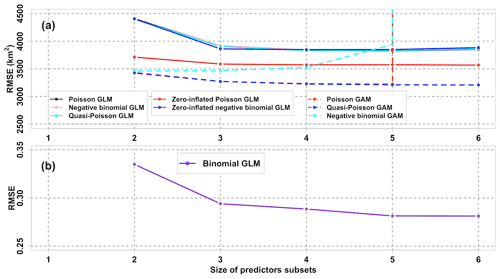Figure 3Comparisons of mean 10-fold CV RMSEs among different regression models with various sizes of predictors subsets. The response variable in (b) binomial GLM and (a) other models is hypoxia occurrence (hypoxia) and hypoxic area (HA), respectively. Note that the CV RMSEs of negative binomial GAM and Poisson GAM with the size of 6 are out of the range shown. CV RMSE curves of the Poisson GLM, negative binomial GLM, and quasi-Poisson GLM overlap, while those of Poisson GAM and quasi-Poisson GAM overlap when the size  5. The minimum size of predictor subsets is 2, since PEA and SOCalt are forced into every subset.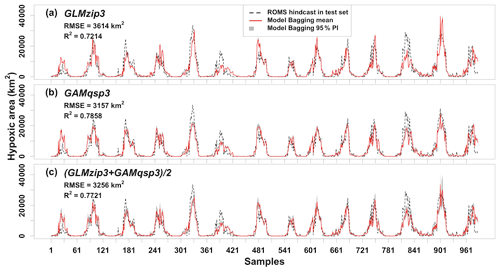Figure 4Comparisons of model-predicted HA and ROMS-hindcast HA in the test set. RMSEs and R2s are derived between the model bagging mean and ROMS-hindcast HA.

Table 2Mean absolute percentage bias between predicted and hindcast HA in the test set within different ranges of hindcast HA. The mean bias when hindcast HA <5000 km2 is not shown, since the prediction accuracy at high HA ranges is a more important feature of HA prediction models. The threshold of 5000 km2 is chosen because it is the goal HA set by the action plan (Mississippi River/Gulf of Mexico Watershed Nutrient Task Force, 2001, 2008). HA above this threshold is more worthy of attention.### 3.2.3 Model interpretation for GLMzip3

We applied the complete ROMS training set to the model construction of GLMzip3. Coefficients for PEA, SOCalt, and DCPTemp (Table 3) are all found to be significantly positive (p<0.001) in the count model, while coefficients for these predictors are significantly negative (p<0.001) in the zero-excess model. The count model simulates HA, while the zero-excess model estimates the probability of HA being zero. Higher PEA is consistent with stronger water stratification, while higher SOCalt and DCPTemp are both corresponding to higher sediment oxygen consumption. Therefore, there is no surprise that higher PEA, SOCalt, and DCPTemp are related to greater HA and higher hypoxia occurrence or a lower probability of HA being zero. Results indicate that GLMzip3 essentially builds up reasonable relationships between the response and predictor variables with a high agreement with physical and biochemical mechanisms. Since the ranges of normalized predictors are from 0 to 1, comparisons of regression coefficients indicate that effects of PEA (2.8037 in the count model and 10.4439 in the zero-excess model, same hereafter) are considered more important than SOCalt (0.9057 and 7.3100) and DCPTemp (0.8425 and 9.5698). The result is consistent with the findings of previous studies which emphasized that the physical impacts are stronger than the biological impacts on HA estimates (Yu et al., 2015; Mattern et al., 2013).

Table 3Regression coefficients of GLMzip3.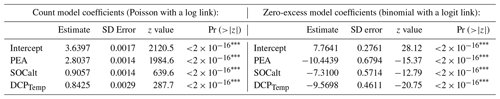Significance codes: ${}^{\ast \ast \ast }$ 0, ${}^{\ast \ast }$ 0.001,  0.01. Log likelihood: $-\mathrm{2.675}×{\mathrm{10}}^{\mathrm{6}}$ on 8 degrees of freedom.

## 3.3 Generalized additive models (GAMs) and the ensemble model

GAMs are explored with an expectation of improving prediction performance in HA peaks by introducing non-parametric effects of predictors. Using function gam in R package mgcv (version 1.8-36; Wood, 2011) with smooth functions as pure thin plate regression splines (degrees of freedom = 9; Wood, 2003), three GAMs are studied and compared, i.e., Poisson GAM, quasi-Poisson GAM, and negative binomial GAM. Following the same procedure in GLM exploration, the best-subset searching approach is applied to the GAMs first. Although mean 10-fold CV RMSEs for the Poisson and quasi-Poisson GAMs (Fig. 3a) exhibit insignificant differences at sizes from 2 to 5, the CV RMSEs for the former increase dramatically at a size of 6, which indicates that the model stability decreases with size. The negative binomial GAM has the greatest mean CV RMSEs among the GAMs studied and has an extremely high mean CV RMSE at the size of 6. The quasi-Poisson GAM is considered the best GAM among the three. Although the mean CV RMSEs for the quasi-Poisson GAM reach the lowest at the size of 6, the best size is considered three (including PEA, SOCalt, and DCPTemp) at which CV RMSEs exhibit the most saline decline and beyond which mean CV RMSEs stabilize around 3200 km2. The quasi-Poisson GAM with three predictors involved is symbolized as GAMqsp3.

Component plots of GAMqsp3 (Fig. 5) imply that HA generally increases as the chosen predictors increase. Note that the summation of all smooth-function terms contributes directly to the log of fitted HA. Such results agree with those found by model GLMzip3. However, the component plots provide more detailed information about the rate of changes in HA. The effective degrees of freedom range from 6.79 to 8.90, indicating strong non-linear effects of the predictors on the variability in HA. HA is more sensitive to the predictors in the low-value ranges but becomes nearly stable in the medium- and high-value ranges of predictors. This implies that bottom hypoxia develops rapidly in early summer when water stratification and sediment oxygen demand start to increase. On the other hand, the smooth functions of SOCalt and DCPTemp have a sharper slope than that of PEA at the low-value range. It suggests that at the first stage of hypoxia development in late spring and early summer, sedimentary biochemical processes contribute more than water stratification. The bottom hypoxic water further extends with a much lower expansion rate as the stratification and SOCalt further intensify. Nevertheless, the smooth function of PEA is slightly greater and also has a more acute slope than those found for SOCalt and DCPTemp in the medium- and high-value regimes of the predictors. It indicates that the HA variability is more related to the hydrodynamic changes in the shelf than the biochemical effects during mid-summers. The result is consistent with the findings by Yu et al. (2015) and Mattern et al. (2013). The GAMqsp3 model provides reasonable interpretations of the mechanisms of hypoxic area.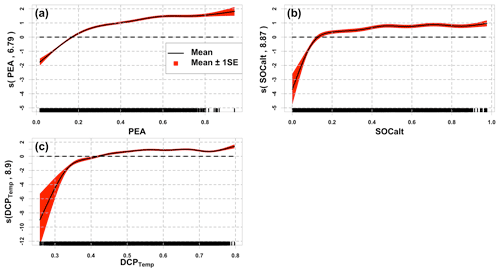Figure 5Component plots of the model GAMqsp3. Solid black lines represent the mean of the smooth function, while the red area denotes the range of mean ±1 SE (standard error). Numbers in brackets represent effective degrees of freedom for the corresponding smooth terms. Black bars at the x axis indicate the density of corresponding normalized predictors. Dashed black lines are straight lines of zero along the predictor domains.

The prediction performance of GAMqsp3 is estimated using the bagging ensemble method (Fig. 4b). The RMSE and R2 between the bagging mean and ROMS-hindcast HA are 3157 km2 and 0.7858, respectively. They are 13 % lower and 9 % higher than the corresponding statistics found for GLMzip3, respectively. MAPB between GAMqsp3 predicted and hindcast HA ranges from 25 % to 40 % with an average of 30 % (Table 2). Such statistics are generally lower than those found in GLMzip3. Results suggest that GAMqsp3 outcompetes GLMzip3 in terms of overall performance. However, GAMqsp3 tends to underestimate HA peaks (such as the peaks around samples 750 and 901), some of which are overestimated by GLMzip3. Therefore, instead of determining the best model out of the two, ensemble HA predictions blending efforts of both GLMzip3 and GAMqsp3 are carried out with an expectation to improve model performance in the peak forecast. We assumed that the contributions of GLMzip3 and GAMqsp3 are equally weighted and thus averaged the predicted HA by GLMzip3 and GAMqsp3 and calculated the 95 % PIs given the bagging results of these models (Fig. 4c). As expected, the overall performance of the ensemble forecast is somewhere between the performance of GLMzip3 and GAMqsp3 with an RMSE of 3256 km2 and an R2 of 0.7721. However, some HA peak events (such as the peaks around samples 750 and 901) which are overestimated by GLMzip3 but are underestimated by GAMqsp3 are accurately predicted by the ensemble approach. MAPB also indicates an increase in peak prediction performance by the ensemble model. The statistic is within a range of 25 % to 36 % with an average of 29 %. At extreme peaks (hindcast HA  30 000 km2), compared to the MAPB by GLMzip3 (29 %) and by GAMqsp3 (28 %), the statistic decreases to 25 % by the ensemble model. The ensemble model provides a higher accuracy in peak forecast given minor sacrifices in overall performance.

## 3.4 Application to global forecast products (HYCOM)

The power of the prediction model relies on the availability of the forecast of predictors. In this section, we discuss the model's transferability using an independent global ocean product. The Global Ocean Forecasting System (GOFS) 3.1 provides global daily analysis products and an 8 d forecast in a daily interval with a horizontal resolution of $\mathrm{1}/\mathrm{12}{}^{\circ }$. The products (hereafter referred to as HYCOM-derived products) are derived by a 41-layer HYCOM global model (Bleck and Boudra, 1981; Bleck, 2002) with data assimilated via the Navy Coupled Ocean Data Assimilation (NCODA) system (Cummings, 2005; Cummings and Smedstad, 2013). The Mississippi River total nitrate + nitrite loadings are provided by USGS NWIS as described in Sect. 2.1.2. Daily HYCOM-derived hydrodynamics and USGS river nitrogen loads from 1 January 2007 to 26 August 2020 are used to reconstruct predictors of PEA, SOCalt, and DCPTemp. Relationships of ROMS-derived and HYCOM-derived predictors are examined in Fig. 6. The magnitudes of HYCOM-derived SOCalt and DCPTemp match up with the corresponding ROMS-derived predictors, respectively, although HYCOM-derived predictors are found to be slightly greater. Simple linear regression for these predictors illustrates that the linear relationships between the ROMS and HYCOM products are significant with the R2 ranging from 0.94 to 0.96. The intercept terms are at least 1 order of magnitude smaller than the magnitudes of corresponding predictors. Therefore, the HYCOM global products are deemed to agree with the ROMS hindcasts for SOCalt and DCPTemp. Nevertheless, the magnitude of HYCOM-derived PEA is found to be much lower than the ROMS-derived PEA (Fig. 6a). Simple linear regression indicates a significant linear relationship between the natural log transformation of PEA from the two datasets (R2=0.66).

At land–sea interfaces, the HYCOM global model is forced by monthly riverine discharges, which weaken the model performance in coastal regions. The hydrodynamics in the LaTex shelf is highly affected by the freshwater and momentum from the Mississippi and the Atchafalaya rivers. Monthly river forcings in HYCOM are essentially weaker than daily forcings used in our ROMS setups and can result in a less stratified water column (i.e., lower PEA). Therefore, it is necessary to scale the magnitude of HYCOM-derived PEA to that of the ROMS hindcast. It can be achieved by using the natural log transformation and simple linear regression as discussed. We then adjusted HYCOM-derived PEA but kept the HYCOM-derived SOCalt and DCPTemp unchanged before the application of the ensemble model.

The bagging approach is implemented again to assess the performances of the ensemble model. During each iteration (N=1000), GLMzip3 and GAMqsp3 are trained using the ROMS training set and then applied to the adjusted HYCOM-derived predictors for HA prediction from 1 January 2012 to 26 August 2020 (Fig. 7a). The ensemble method provides averages and 95 % PIs of predicted HA blending bagging results by GLMzip3 and GAMqsp3. Compared to observed HA by mid-summer shelf-wide cruises, the ensemble model fails in the summers of 2013, 2014, 2017, and 2018 but provides accurate predictions in other summers. The width of 95 % PI is larger during high-HA periods, suggesting less stability in the HA peak forecast. The overall performance is barely acceptable, with an R2 of 0.4242, an RMSE of 5088 km2, and an SI of 38 %. The bias against the observations can be ascribed to HYCOM's failures in reproducing the shelf hydrodynamics, although HYCOM-derived predictors are adjusted before being applied to the model (Fig. 6a). We noticed that among the three variables, HYCOM-derived PEA exhibits the largest deviation from that generated by ROMS. We then applied the model using ROMS-derived PEA, HYCOM-derived SOCalt, and HYCOM-derived DCPTemp (Fig. 7b). The performance of the ensemble model was largely enhanced with a higher R2 (0.9255), a much lower RMSE (3751 km2), and a lower SI (28 %) compared to the one using the pure HYCOM products. These results indicate that the ensemble model can produce a highly accurate prediction for HA summer peaks once water stratification is well resolved. Instead of using monthly river forcings, the HYCOM model may possibly resolve the shelf hydrodynamics by utilizing daily river discharges of the Mississippi and the Atchafalaya rivers.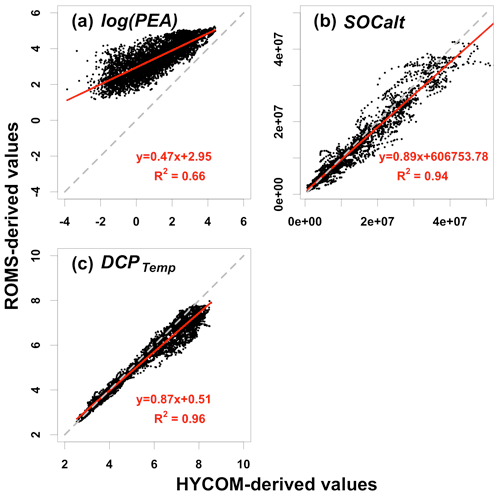Figure 6Scatterplots of (a) log(PEA) (unit: log(J m−3)), (b) SOCalt (unit: mmol s−1), and (c) DCPTemp (unit: non-dim) between ROMS and HYCOM simulations. Note that the solid red lines represent linear regression lines, while the dashed grey lines are diagonals with a slope of 1 and an intercept of 0. Daily data compared are from 2007 to 2020.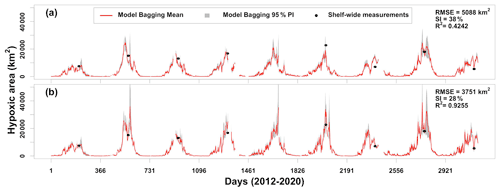Figure 7Comparisons of daily predicted HA by ensemble model ((GLMzip3 + GAMqsp3) $/$ 2) when applied to adjusted HYCOM products and shelf-wide measurements from 2012 to 2020. Model results shown in (a) are predicted using pure HYCOM-derived products (i.e., PEA, SOCalt, and DCPTemp), while those in (b) are predicted by ROMS-derived PEA, HYCOM-derived SOCalt, and HYCOM-derived DCPTemp. Discontinuity of the predictions is due to the lack of riverine nitrate + nitrite records at USGS site 07374000 in the Mississippi River.

4 Discussion

## 4.1 Model performance evaluation

To further assess the robustness of our model, we reviewed a suite of existing forecast models that are transitioned operationally (in early June) to the NOAA ensemble forecast for each summer (data sources are listed in Table 4). Using the ROMS-derived predictors, daily HA predictions during the shelf-wide cruise periods are averaged for each summer from 2012 to 2020 and are compared to the cruise observations. As shown in Fig. 8a, our model predictions fit well with the shelf-wide observation for summers with or without strong windy events prior to the cruises. Other seasonal forecast models have similar performances to our model in fair-weather summers (i.e., 2012, 2014, 2015, and 2017) but fail to produce an accurate forecast for several summers with strong wind conditions (i.e., 2018 and 2020). Percentage differences between predictions and observations (Fig. 8b) also emphasize the superiority of our model with the percentages ranging from 24 % to 7 % for fair-weather summers and from 7 % to 35 % for summers with strong winds or storms. All models either underestimate or overestimate observed HA in fair-weather summers but overestimate HA in windy summers. Our model provides the most accurate overall performance with the highest R2 (0.9200, N=8), the lowest RMSE (2005 km2, N=8), the lowest SI (15 %, N=8), and the lowest MAPB (18 %, N=8) among all models (Table 4). The multiple linear regression model developed by Forrest et al. (2011) provides the second best prediction. For fair-weather summers, the NOAA ensemble predictions produce the best estimation of the observed HA with an MAPB of 9 % (N=4), while our model results rank the second (15 %, N=4). However, our model performs the best in windy summers with an MAPB of 18 % (N=4), while other models produce an MAPB from 33 % to 74 %.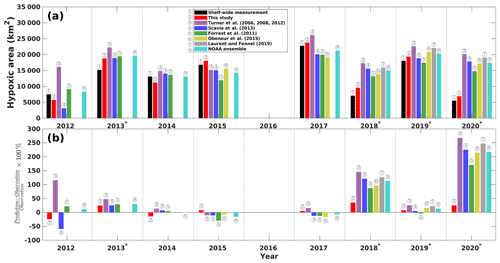Figure 8(a) Comparisons of shelf-wide measured and the best estimates of model-predicted HA during the shelf-wide cruise periods. (b) Percentage differences between different model predictions and shelf-wide measurements. The superscript asterisks indicate high-wind years prior to the cruises.

Models developed by Turner et al. (2006, 2008, 2012) and Laurent and Fennel (2019) are calibrated on May nitrate or nitrate + nitrite loads from the Mississippi–Atchafalaya River basin, assuming that the predicted HA in summers is under fair weather. It is expected that models excluding wind effects can hardly produce accurate forecasts during summers with strong winds or storms. Wind mixing effects on HA are considered in reaeration by introducing a wind stress term in the mechanistic model (Obenour et al., 2015), while in the Bayesian model by Scavia et al. (2013), the wind effects are considered indirectly via an estimation based on current velocity and the reaeration rate given different wind conditions (i.e., fair weather, strong westerly winds, and storms). However, as shown in Fig. 1a, PEAwind, which can also be interpreted as wind power, is found poorly correlated to daily HA ($R=-\mathrm{0.2458}$) compared to other highly correlated predictors and is dropped out of the candidate list by the best-subset searching approach. Forrest et al. (2011) also found that monthly wind power is not significantly correlated to summer HA due to the short timescales of strong wind events. Therefore, the wind mixing effects considered by Obenour et al. (2015) and Scavia et al. (2013) have limited contribution to the prediction of the interannual variability in HA. Indeed, our model construction process indicates that wind mixing, freshwater plume, and water temperature jointly control the water stratification and vertical mixing, which directly modulates the reoxygenation of shelf water. PEA can serve better in representing such effects rather than by wind speed or wind power alone. The daily PEA is significantly correlated to daily HA (R=0.8178, p<0.01; Fig. 1a), while the non-linear effects of PEA cannot be neglected (Fig. 5a). Therefore, an accurate forecast of shelf hydrodynamics is critical for a robust summer HA prediction.

Table 4Statistics comparisons between model predictions and the shelf-wide measurements. The R2 values for predictions by Obenour et al. (2015) and Laurent and Fennel (2019) are not given, since the numbers of available records are small (N=5 and 3, respectively). Numbers in parentheses indicate the numbers of compared records. Subscript “fair” and “windy” indicate that averages of corresponding statistics are conducted for fair-weather and windy summers, respectively.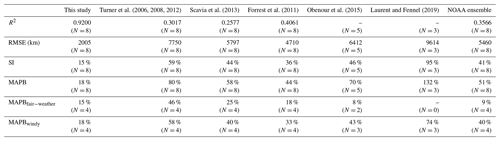Data source (last access in 15 June 2022): https://gulfhypoxia.net/ (Turner et al., 2006, 2008, 2012), http://scavia.seas.umich.edu/hypoxia-forecasts/ (Scavia et al., 2013), https://www.vims.edu/research/topics/dead_zones/forecasts/gom/index.php (Forrest et al., 2011), https://obenour.wordpress.ncsu.edu/news/ (Obenour et al., 2015), https://memg.ocean.dal.ca/news/ (Laurent and Fennel, 2019), https://www.noaa.gov/news (NOAA ensemble).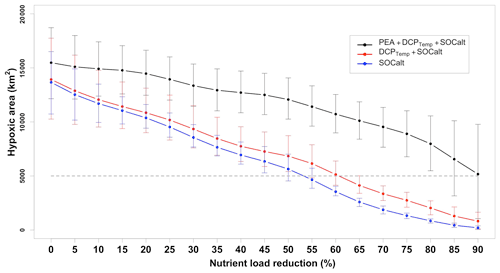Figure 9Mean for 2015–2020 (except 2016) predicted HA in scenarios of different nutrient load reduction strategies given different sets of predictors considered. Predictions by the ensemble model are conducted individually for the shelf-wide cruise periods in different summers and averaged from 2015–2020. Horizontal bars indicate ranges of 95 % PIs. Grey dashed lines represent the goal of 5000 km2 set by the Mississippi River/Gulf of Mexico Hypoxia Task Force. Note here nutrient reduction percentages refer to mid-June nutrient loads in corresponding years.

## 4.2 Task force nutrient reduction

The recommended reduction strategy of our model is much more demanding than that of other models (Scavia et al., 2013; Obenour et al., 2015; Turner et al., 2012; Laurent and Fennel, 2019), which recommend a load reduction of 52 %–58 % related to the 1980–1996 average (Scavia et al., 2017). A recommendation of 92 % reduction is close to that by Forrest et al. (2011) (80 %) when the coastal westerlies from 15 June to 15 July were considered in their regression model. Since water stratification is attributed to not only wind mixing effects but also effects from other physical processes (e.g., riverine freshwater transports and surface heating), models developed based solely on May nutrient loads (Turner et al., 2012; Laurent and Fennel, 2019) or nutrient loads and wind mixing (Scavia et al., 2013; Obenour et al., 2015) fail to capture water stratification's contribution to hypoxia development. If a model considers the variability in HA to rely highly on the nutrient loads, then a moderate decrease in nutrient loads would result in a substantial HA reduction. For further illustration, we reran the model without consideration of the PEA (i.e., using DCPTemp and SOCalt or using only SOCalt). Model results show a substantial shrinking of HA with moderately reduced riverine nitrogen loads (Fig. 9). In details, if only DCPTemp and SOCalt are used as the predictors, a nutrient reduction of 60 % will satisfy the 5000 km2 HA goal. And if we only use SOCalt as the predictor, then a 55 % reduction is sufficient. These results highlight the importance of considering PEA in HA predictions.

5 Conclusions

In this study, we present a novel HA forecast model for the LaTex shelf using statistical analysis. The model is trained using numeric simulations from 1 January 2007 to 26 August 2020 by a three-dimensional coupled hydrodynamic–biogeochemical model (ROMS). Multiple GLMs (regular Poisson GLMs, quasi-Poisson GLMs, negative binomial GLMs, zero-inflated Poisson GLMs, and zero-inflated negative binomial GLMs) and GAMs (regular Poisson GAMs, quasi-Poisson GAMs, and regular negative binomial GAMs) are assessed for HA predictions. Comparisons of model prediction performance illustrate that an ensemble model combining the prediction efforts of a zero-inflated Poisson GLM (GLMzip3) and a quasi-Poisson GAM (GAMqsp3) provides the most accurate HA forecast using PEA, SOCalt, and DCPTemp as predictors. The ensemble model is capable of explaining up to 77 % of the total variability in the hindcast HA and also provides a low RMSE of 3256 km2 and low MAPBs for overall (29 %) and peak predictions (25 %) when compared to the daily ROMS hindcasts.

We then applied the hydrodynamics field generated by a global model (HYCOM, GOFS 3.1) and performed a HA hindcast for the period from 1 January 2012 to 26 August 2020. The overall performance is barely acceptable with an R2 of 0.4242, an RMSE of 5088 km2, and an SI of 38 % against the shelf-wide summer cruise observations, largely due to HYCOM's relatively poor representation of shelf stratification. A substitution of ROMS-derived PEA led to a pronounced improvement with an R2 of 0.9255, an RMSE of 3751 km2, and an SI of 28 %.

The ensemble model also provides an efficient yet more robust summer HA forecast compared to existing HA forecast models. Comparing against the shelf-wide cruise observations, our model provides a high R2 (0.9200 vs. 0.2577–0.4061 by existing forecast models, same comparison hereinafter); a low RMSE (2005 km2 vs. 4710–9614 km2); a low SI (15 % vs. 36 %–95 %); and low MAPBs for overall (18 % vs. 44 %–132 %), fair-weather summer (15 % vs. 8 %–46 %), and windy-summer (18 % vs. 33 %–74 %) predictions. Sensitivity tests were conducted and suggest that a 92 % reduction in riverine nutrients related to the 1980–1996 summer average is required to meet the goal of a 5000 km2 HA. These results highlight the importance of considering PEA in HA prediction.

Appendix A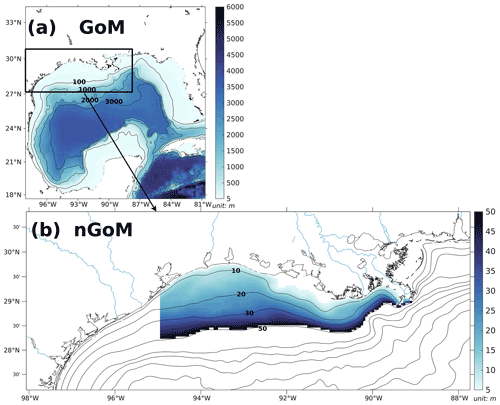Figure A1(a) Bathymetry of the entire domain of the Gulf–COAWST described in the accompanying study (Part 1) and (b) zoom-in bathymetry plot of the northern Gulf of Mexico (nGoM). The range of bathymetry of the color shaded area in (b) is from 6 to 50 m, over which the regional averages of parameters are conducted.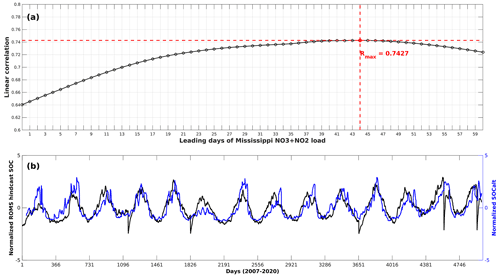Figure A3(a) Lead–lag correlation coefficients between ROMS-hindcast daily SOC and SOCalt (Mississippi River inorganic nitrogen loads ${e}^{\mathrm{0.0693}{T}_{\mathrm{b}}}$) with the Mississippi nitrogen loads leading by different days; (b) daily time series of ROMS-hindcast SOC and SOCalt when the Mississippi nitrogen loads lead by 44 d. The time series are regional average results over the LaTex shelf and are normalized.

Table A1A correlation matrix of monthly mean inorganic nutrient loads by the Mississippi River and the Atchafalaya River from 2007 to 2020. Correlation coefficients shown are all significant (p<0.001).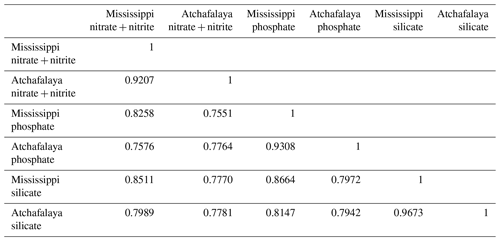Code and data availability

Model data are available at the Louisiana State University (LSU) mass storage system, and details are on the web page of the Coupled Ocean Modeling Group at LSU (https://lsu.app.box.com/folder/168361434653?s=8qhpz2glpxlbsu9z9m6g4cudcegfseh4, Ou, 2022). Data requests can be sent to the corresponding author via this web page.

Author contributions

BL and ZGX designed the experiments, and YO carried them out. YO developed the model code and performed the simulations. YO, BL, and ZGX prepared the manuscript.

Competing interests

The contact author has declared that none of the authors has any competing interests.

Disclaimer

Publisher's note: Copernicus Publications remains neutral with regard to jurisdictional claims in published maps and institutional affiliations.

Acknowledgements

Research support was provided through the Bureau of Ocean Energy Management (grant nos. M17AC00019 and M20AC10001). We thank Jerome Fiechter at the University of California, Santa Cruz, for sharing his NEMURO (North Pacific Ecosystem Model for Understanding Regional Oceanography) model codes. Computational support was provided by the high-performance computing facility (clusters SuperMIC and QueenBee3) at Louisiana State University.

Financial support

This research has been supported by the Bureau of Ocean Energy Management (grant nos. M17AC00019 and M20AC10001).

Review statement

This paper was edited by Tina Treude and reviewed by Brandon Jarvis and two anonymous referees.

References

Battaglin, W. A., Aulenbach, B. T., Vecchia, A., and Buxton, H. T.: Changes in streamflow and the flux of nutrients in the Mississippi-Atchafalaya River Basin, USA, 1980–2007, Scientific Investigations Report, Reston, VA, U.S. Geological Survey, https://doi.org/10.3133/sir20095164, 2010.

Bianchi, T. S., DiMarco, S. F., Cowan, J. H., Hetland, R. D., Chapman, P., Day, J. W., and Allison, M. A.: The science of hypoxia in the northern Gulf of Mexico: A review, Sci. Total Environ., 408, 1471–1484, https://doi.org/10.1016/j.scitotenv.2009.11.047, 2010.

Bleck, R.: An oceanic general circulation model framed in hybrid isopycnic-Cartesian coordinates, Ocean Model., 4, 55–88, https://doi.org/10.1016/S1463-5003(01)00012-9, 2002.

Bleck, R. and Boudra, D. B.: Initial testing of a numerical ocean circulation model using a hybrid (quasi-isopycnic) vertical coordinate, J. Phys. Oceanogr., 11, 755–770, https://doi.org/10.1175/1520-0485(1981)011<0755:ITOANO>2.0.CO;2, 1981.

Chesney, E. J. and Baltz, D. M.: The effects of hypoxia on the northern Gulf of Mexico Coastal Ecosystem: A fisheries perspective, in: Coastal Hypoxia: Consequences for Living Resources and Ecosystems, Am. Geophys. Union, 58, 321–354, https://doi.org/10.1029/CE058p0321, 2001.

Conley, D. J., Paerl, H. W., Howarth, R. W., Boesch, D. F., Seitzinger, S. P., Havens, K. E., Lancelot, C., and Likens, G. E.: Controlling Eutrophication: Nitrogen and Phosphorus, Science, 323, 1014–1015, https://doi.org/10.1126/science.1167755, 2009.

Craig, J. K.: Aggregation on the edge: Effects of hypoxia avoidance on the spatial distribution of brown shrimp and demersal fishes in the Northern Gulf of Mexico, Mar. Ecol. Prog. Ser., 445, 75–95, https://doi.org/10.3354/meps09437, 2012.

Craig, J. K. and Bosman, S. H.: Small Spatial Scale Variation in Fish Assemblage Structure in the Vicinity of the Northwestern Gulf of Mexico Hypoxic Zone, Estuar. Coast., 36, 268–285, https://doi.org/10.1007/s12237-012-9577-9, 2013.

Craig, J. K. and Crowder, L. B.: Hypoxia-induced habitat shifts and energetic consequences in Atlantic croaker and brown shrimp on the Gulf of Mexico shelf, Mar. Ecol. Prog. Ser., 294, 79–94, https://doi.org/10.3354/meps294079, 2005.

Cummings, J. A.: Operational multivariate ocean data assimilation, Q. J. R. Meteorol. Soc., 131, 3583–3604, https://doi.org/10.1256/qj.05.105, 2005.

Cummings, J. A. and Smedstad, O. M.: Variational Data Assimilation for the Global Ocean, in: Data Assimilation for Atmospheric, Oceanic and Hydrologic Applications, Vol. 2, edited by: Park, S. K. and Xu, L., Springer Berlin Heidelberg, 303–343, https://doi.org/10.1007/978-3-642-35088-7_13, 2013.

de Mutsert, K., Steenbeek, J., Lewis, K., Buszowski, J., Cowan, J. H., and Christensen, V.: Exploring effects of hypoxia on fish and fisheries in the northern Gulf of Mexico using a dynamic spatially explicit ecosystem model, Ecol. Modell., 331, 142–150, https://doi.org/10.1016/j.ecolmodel.2015.10.013, 2016.

Feng, Y., Fennel, K., Jackson, G. A., DiMarco, S. F., and Hetland, R. D.: A model study of the response of hypoxia to upwelling-favorable wind on the northern Gulf of Mexico shelf, J. Mar. Syst., 131, 63–73, https://doi.org/10.1016/j.jmarsys.2013.11.009, 2014.

Fennel, K., Hetland, R., Feng, Y., and DiMarco, S.: A coupled physical-biological model of the Northern Gulf of Mexico shelf: model description, validation and analysis of phytoplankton variability, Biogeosciences, 8, 1881–1899, https://doi.org/10.5194/bg-8-1881-2011, 2011.

Fennel, K., Hu, J., Laurent, A., Marta-Almeida, M., and Hetland, R.: Sensitivity of hypoxia predictions for the northern Gulf of Mexico to sediment oxygen consumption and model nesting, J. Geophys. Res.-Ocean., 118, 990–1002, 2013.

Fennel, K., Laurent, A., Hetland, R., Justic, D., Ko, D. S., Lehrter, J., Murrell, M., Wang, L., Yu, L., and Zhang, W.: Effects of model physics on hypoxia simulations for the northern Gulf of Mexico Mean for 2015: A model intercomparison, J. Geophys. Res.-Ocean., 121, 5731–5750, https://doi.org/10.1002/2015JC011516, 2016.

Forrest, D. R., Hetland, R. D., and DiMarco, S. F.: Multivariable statistical regression models of the areal extent of hypoxia over the Texas-Louisiana continental shelf, Environ. Res. Lett., 6, 045002, https://doi.org/10.1088/1748-9326/6/4/045002, 2011.

Del Giudice, D., Matli, V. R. R., and Obenour, D. R.: Bayesian mechanistic modeling characterizes Gulf of Mexico hypoxia: 1968–2016 and future scenarios, Ecol. Appl., 30, 1–14, https://doi.org/10.1002/eap.2032, 2020.

Hazen, E. L., Craig, J. K., Good, C. P., and Crowder, L. B.: Vertical distribution of fish biomass in hypoxic waters on the gulf of Mexico shelf, Mar. Ecol. Prog. Ser., 375, 195–207, https://doi.org/10.3354/meps07791, 2009.

Hetland, R. D. and DiMarco, S. F.: How does the character of oxygen demand control the structure of hypoxia on the Texas-Louisiana continental shelf?, J. Mar. Syst., 70, 49–62, https://doi.org/10.1016/j.jmarsys.2007.03.002, 2008.

Jackman, S.: pscl: Classes and Methods for R Developed in the Political Science Computational Laboratory, https://github.com/atahk/pscl/ (last access: 7 September 2021), 2020.

Justić, D. and Wang, L.: Assessing temporal and spatial variability of hypoxia over the inner Louisiana-upper Texas shelf: Application of an unstructured-grid three-dimensional coupled hydrodynamic-water quality model, Cont. Shelf Res., 72, 163–179, https://doi.org/10.1016/j.csr.2013.08.006, 2014.

Katin, A., Del Giudice, D., and Obenour, D. R.: Temporally resolved coastal hypoxia forecasting and uncertainty assessment via Bayesian mechanistic modeling, Hydrol. Earth Syst. Sci., 26, 1131–1143, https://doi.org/10.5194/hess-26-1131-2022, 2022.

LaBone, E. D., Rose, K. A., Justic, D., Huang, H., and Wang, L.: Effects of spatial variability on the exposure of fish to hypoxia: a modeling analysis for the Gulf of Mexico, Biogeosciences, 18, 487–507, https://doi.org/10.5194/bg-18-487-2021, 2021.

Lambert, D.: Zero-inflated poisson regression, with an application to defects in manufacturing, Technometrics, 34, 1–14, 1992.

Laurent, A. and Fennel, K.: Time-Evolving, Spatially Explicit Forecasts of the Northern Gulf of Mexico Hypoxic Zone, Environ. Sci. Technol., 53, 14449–14458, https://doi.org/10.1021/acs.est.9b05790, 2019.

Laurent, A., Fennel, K., Ko, D. S., and Lehrter, J.: Climate change projected to exacerbate impacts of coastal Eutrophication in the Northern Gulf of Mexico, J. Geophys. Res.-Ocean., 123, 3408–3426, https://doi.org/10.1002/2017JC013583, 2018.

Matli, V. R. R., Fang, S., Guinness, J., Rabalais, N. N., Craig, J. K., and Obenour, D. R.: Space-Time Geostatistical Assessment of Hypoxia in the Northern Gulf of Mexico, Environ. Sci. Technol., 52, 12484–12493, https://doi.org/10.1021/acs.est.8b03474, 2018.

Mattern, J. P., Fennel, K., and Dowd, M.: Sensitivity and uncertainty analysis of model hypoxia estimates for the Texas-Louisiana shelf, J. Geophys. Res.-Ocean., 118, 1316–1332, https://doi.org/10.1002/jgrc.20130, 2013.

McCarthy, M. J., Carini, S. A., Liu, Z., Ostrom, N. E., and Gardner, W. S.: Oxygen consumption in the water column and sediments of the northern Gulf of Mexico hypoxic zone, Estuar. Coast. Shelf Sci., 123, 46–53, https://doi.org/10.1016/j.ecss.2013.02.019, 2013.

Mississippi River/Gulf of Mexico Watershed Nutrient Task Force: Action Plan for Reducing, Mitigating, and Controlling Hypoxia in the Northern Gulf of Mexico, Washington, DC, US Environmental Protection Agency, https://www.epa.gov/sites/default/files/2015-03/documents/2001_04_04_msbasin_actionplan2001.pdf (last access: 29 July 2022), 2001.

Mississippi River/Gulf of Mexico Watershed Nutrient Task Force: Gulf Hypoxia Action Plan 2008 for Reducing, Mitigating, and Controlling Hypoxia in the Northern Gulf of Mexico and Improving Water Quality in the Mississippi River Basin, Washington, DC, US Environmental Protection Agency, https://www.epa.gov/sites/default/files/2015-03/documents/2008_8_28_msbasin_ghap2008_update082608.pdf (last access: 29 July 2022), 2008.

Monteith, J. and Unsworth, M.: Principles of environmental physics: plants, animals, and the atmosphere, 4th Edn., Academic Press, https://doi.org/10.1016/C2010-0-66393-0, 2014.

Murray, F. W.: On the Computation of Saturation Vapor Pressure, J. Appl. Meteorol. Climatol., 6, 203–204, https://doi.org/10.1175/1520-0450(1967)006<0203:OTCOSV>2.0.CO;2, 1967.

Murrell, M. C. and Lehrter, J. C.: Sediment and Lower Water Column Oxygen Consumption in the Seasonally Hypoxic Region of the Louisiana Continental Shelf, Estuar. Coast., 34, 912–924, https://doi.org/10.1007/s12237-010-9351-9, 2011.

Obenour, D. R., Michalak, A. M., and Scavia, D.: Assessing biophysical controls on Gulf of Mexico hypoxia through probabilistic modeling, Ecol. Appl., 25, 492–505, https://doi.org/10.1890/13-2257.1, 2015.

Ou, Y.: Data for Hydrodynamic and biochemical impacts on the development of hypoxia in the Louisiana–Texas shelf – Part 2: statistical modeling and hypoxia prediction, LSU [data set], https://lsu.app.box.com/folder/168361434653?s=8qhpz2glpxlbsu9z9m6g4cudcegfseh4, last access: 26 July 2022.

Picard, A., Davis, R. S., Gläser, M., and Fujii, K.: Revised formula for the density of moist air (CIPM-2007), Metrologia, 45, 149–155, https://doi.org/10.1088/0026-1394/45/2/004, 2008.

Purcell, K. M., Craig, J. K., Nance, J. M., Smith, M. D., and Bennear, L. S.: Fleet behavior is responsive to a large-scale environmental disturbance: Hypoxia effects on the spatial dynamics of the northern Gulf of Mexico shrimp fishery, PLoS One, 12, e0183032, https://doi.org/10.1371/journal.pone.0183032, 2017.

Rabalais, N. N. and Baustian, M. M.: Historical Shifts in Benthic Infaunal Diversity in the Northern Gulf of Mexico since the Appearance of Seasonally Severe Hypoxia, Diversity, 12, 49, https://doi.org/10.3390/d12020049, 2020.

Rabalais, N. N. and Turner, R. E.: Gulf of Mexico Hypoxia: Past, Present, and Future, Limnol. Oceanogr. Bull., 28, 117–124, https://doi.org/10.1002/lob.10351, 2019.

Rabalais, N. N., Turner, R. E., and Wiseman, W. J.: Gulf of Mexico hypoxia, a.k.a. “The dead zone,” Annu. Rev. Ecol. Syst., 33, 235–263, https://doi.org/10.1146/annurev.ecolsys.33.010802.150513, 2002.

Rabalais, N. N., Turner, R. E., Sen Gupta, B. K., Boesch, D. F., Chapman, P., and Murrell, M. C.: Hypoxia in the northern Gulf of Mexico: Does the science support the plan to reduce, mitigate, and control hypoxia?, Estuar. Coast., 30, 753–772, https://doi.org/10.1007/BF02841332, 2007a.

Rabalais, N. N., Turner, R. E., Gupta, B. K. S., Platon, E., and Parsons, M. L.: Sediments tell the history of eutrophication and hypoxia in the northern Gulf of Mexico, Ecol. Appl., 17, 129–143, https://doi.org/10.1890/06-0644.1, 2007b.

Rabotyagov, S. S., Kling, C. L., Gassman, P. W., Rabalais, N. N., and Turner, R. E.: The economics of dead zones: Causes, impacts, policy challenges, and a model of the gulf of Mexico Hypoxic Zone, Rev. Environ. Econ. Policy, 8, 58–79, https://doi.org/10.1093/reep/ret024, 2014.

Reyes, B. A., Pendergast, J. S., and Yamazaki, S.: Mammalian peripheral circadian oscillators are temperature compensated, J. Biol. Rhythms, 23, 95–98, https://doi.org/10.1177/0748730407311855, 2008.

Saha, S., Moorthi, S., Pan, H.-L., Wu, X., Wang, J., Nadiga, S., Tripp, P., Kistler, R., Woollen, J., Behringer, D., Liu, H., Stokes, D., Grumbine, R., Gayno, G., Wang, J., Hou, Y.-T., Chuang, H. Y., Juang, H.-M. H., Sela, J., Iredell, M., Treadon, R., Kleist, D., Van Delst, P., Keyser, D., Derber, J., Ek, M., Meng, J., Wei, H., Yang, R., Lord, S., van den Dool, H., Kumar, A., Wang, W., Long, C., Chelliah, M., Xue, Y., Huang, B., Schemm, J.-K., Ebisuzaki, W., Lin, R., Xie, P., Chen, M., Zhou, S., Higgins, W., Zou, C.-Z., Liu, Q., Chen, Y., Han, Y., Cucurull, L., Reynolds, R. W., Rutledge, G., and Goldberg, M.: NCEP Climate Forecast System Reanalysis (CFSR) 6-hourly Products, January 1979 to December 2010, Research Data Archive at the National Center for Atmospheric Research, Computational and Information Systems Laboratory [data set], https://doi.org/10.5065/D69K487J, 2010.

Saha, S., Moorthi, S., Wu, X., Wang, J., Nadiga, S., Tripp, P., Behringer, D., Hou, Y.-T., Chuang, H., Iredell, M., Ek, M., Meng, J., Yang, R., Mendez, M. P., van den Dool, H., Zhang, Q., Wang, W., Chen, M., and Becker, E.: NCEP Climate Forecast System Version 2 (CFSv2) 6-hourly Products, Research Data Archive at the National Center for Atmospheric Research, Computational and Information Systems Laboratory [data set], https://doi.org/10.5065/D61C1TXF, 2011.

Scavia, D., Evans, M. A., and Obenour, D. R.: A scenario and forecast model for gulf of mexico hypoxic area and volume, Environ. Sci. Technol., 47, 10423–10428, https://doi.org/10.1021/es4025035, 2013.

Scavia, D., Bertani, I., Obenour, D. R., Turner, R. E., Forrest, D. R., and Katin, A.: Ensemble modeling informs hypoxia management in the northern Gulf of Mexico, P. Natl. Acad. Sci. USA, 114, 8823–8828, https://doi.org/10.1073/pnas.1705293114, 2017.

Siegel, A. F. and Wagner, M. R.: Chapter 12 – Multiple Regression: Predicting One Variable From Several Others, in: Practical Business Statistics, edited by: Siegel, A. F. and Wagner, M. R., Academic Press, London, 371–431, https://doi.org/10.1016/B978-0-12-820025-4.00012-9, 2022.

Simpson, J. H.: The shelf-sea fronts: implications of their existence and behaviour, Philos. T. R. Soc. Lond. Ser. A, 302, 531–546, https://doi.org/10.1098/rsta.1981.0181, 1981.

Simpson, J. H. and Bowers, D.: Models of stratification and frontal movement in shelf seas, Deep-Sea Res. Pt. A, 28, 727–738, https://doi.org/10.1016/0198-0149(81)90132-1, 1981.

Simpson, J. H. and Hunter, J. R.: Fronts in the Irish Sea, Nature, 250, 404–406, https://doi.org/10.1038/250404a0, 1974.

Simpson, J. H., Allen, C. M., and Morris, N. C. G.: Fronts on the Continental Shelf, J. Geophys. Res., 83, 4607–4614, https://doi.org/10.1029/JC083iC09p04607, 1978.

Smith, M. D., Asche, F., Bennear, L. S., and Oglend, A.: Spatial-dynamics of hypoxia and fisheries: The case of Gulf of Mexico brown shrimp, Mar. Resour. Econ., 29, 111–131, https://doi.org/10.1086/676826, 2014.

Turner, R. E., Rabalais, N. N., and Justic, D.: Predicting summer hypoxia in the northern Gulf of Mexico: Riverine N, P, and Si loading, Mar. Pollut. Bull., 52, 139–148, https://doi.org/10.1016/j.marpolbul.2005.08.012, 2006.

Turner, R. E., Rabalais, N. N., and Justic, D.: Gulf of Mexico hypoxia: Alternate states and a legacy, Environ. Sci. Technol., 42, 2323–2327, https://doi.org/10.1021/es071617k, 2008.

Turner, R. E., Rabalais, N. N., and Justić, D.: Predicting summer hypoxia in the northern Gulf of Mexico: Redux, Mar. Pollut. Bull., 64, 319–324, https://doi.org/10.1016/j.marpolbul.2011.11.008, 2012.

van 't Hoff, J. H. and Lehfeldt, R. A.: Lectures in theoretical and physical chemistry: Part I: Chemical dynamics, London: Edward Arnold, London, Edward Arnold, London, OCLC number 220605730, 1899.

Venables, W. N. and Ripley, B. D.: Modern Applied Statistics with S, Fourth, Springer, New York, https://doi.org/10.1007/978-0-387-21706-2, 2002.

Wang, L. and Justić, D.: A modeling study of the physical processes affecting the development of seasonal hypoxia over the inner Louisiana-Texas shelf: Circulation and stratification, Cont. Shelf Res., 29, 1464–1476, https://doi.org/10.1016/j.csr.2009.03.014, 2009.

Warner, J. C., Armstrong, B., He, R., and Zambon, J. B.: Development of a Coupled Ocean-Atmosphere-Wave-Sediment Transport (COAWST) Modeling System, Ocean Model., 35, 230–244, https://doi.org/10.1016/j.ocemod.2010.07.010, 2010.

Wood, S. N.: Thin plate regression splines, J. R. Stat. Soc. Ser. B, 65, 95–114, https://doi.org/10.1111/1467-9868.00374, 2003.

Wood, S. N.: Fast stable restricted maximum likelihood and marginal likelihood estimation of semiparametric generalized linear models, J. R. Stat. Soc. Ser. B, 73, 3–36, https://doi.org/10.1111/j.1467-9868.2010.00749.x, 2011.

Yu, L., Fennel, K., and Laurent, A.: A modeling study of physical controls on hypoxia generation in the northern Gulf of Mexico, J. Geophys. Res.-Ocean., 120, 5019–5039, https://doi.org/10.1002/2014JC010634, 2015.

Zambresky, L.: A verification study of the global WAM model, December 1987–November 1988, ECMWF, Shinfield Park, Reading, https://www.ecmwf.int/node/13201 (last access: 29 July 2022), 1989.

Zeileis, A., Kleiber, C., and Jackman, S.: Regression Models for Count Data in R, J. Stat. Softw., 27, 1–25, https://doi.org/10.18637/jss.v027.i08, 2008.